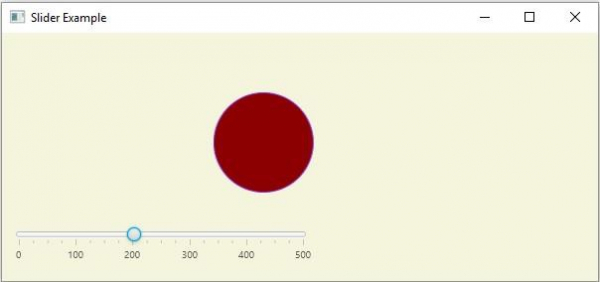# How to set action to a slider using JavaFX?

JavaFX provides a class known as Slider, this represents a slider component which displays continuous range of values. This contains a track on which the number values are displayed. Along the track there is a thumb pointing to the numbers. You can provide the maximum, minimum and initial values of the slider.

## Setting action to the slider

The property of the slider class named value, represents the current value of the slider, the valueProperty() returns a property object representing the current value of the slider. You can set action when the value is changed, by adding listener to this property, using the addListener() method.

## Example

In the following JavaFX example, we have created a slider and a circle.

We have instantiated the Translate (transformation) class and linked the slider new value to the translate value along the x axis and added the translate transform to the circle.

import javafx.application.Application;
import javafx.beans.value.ChangeListener;
import javafx.beans.value.ObservableValue;
import javafx.geometry.Insets;
import javafx.scene.Scene;
import javafx.scene.control.Slider;
import javafx.scene.layout.VBox;
import javafx.scene.paint.Color;
import javafx.scene.shape.Circle;
import javafx.scene.transform.Translate;
import javafx.stage.Stage;
public class SliderAction extends Application {
@Override
public void start(Stage stage) {
//Drawing a Rectangle
Circle circle = new Circle(50);
circle.setFill(Color.DARKRED);
circle.setStroke(Color.BLUEVIOLET);
//Setting the slider
Slider slider = new Slider(0, 500, 0);
slider.setShowTickLabels(true);
slider.setShowTickMarks(true);
slider.setMajorTickUnit(100);
slider.setBlockIncrement(50);
//Setting the width of the slider
slider.setMaxWidth(300);
//Creating the translation transformation
Translate translate = new Translate();
//Linking the transformation to the slider
public void changed(ObservableValue <?extends Number>observable, Number oldValue, Number newValue){
translate.setX((double) newValue);
translate.setY(50);
translate.setZ(100);
}
});
//Adding the transformation to the circle
//VBox to arrange circle and the slider
VBox vbox = new VBox(85);
vbox.setStyle("-fx-background-color: BEIGE");
//Preparing the scene
Scene scene = new Scene(vbox, 600, 250);
stage.setTitle("Slider Example");
stage.setScene(scene);
stage.show();
}
public static void main(String args[]){
launch(args);
}
}

## Output

On executing the above program, it will generate a JavaFX window with a slider and a circle on it. If you move the slider the circle will slide along the x-axis.